# Poplar

How tall is a poplar by the river if we know that 1/5 of its total height is a trunk, 1/10th of the height is the root, and 35m from the trunk to the top of the poplar?

x =  50 m

### Step-by-step explanation:

35 + x/5+x/10 = x

7x = 350

x = 50

Our simple equation calculator calculates it.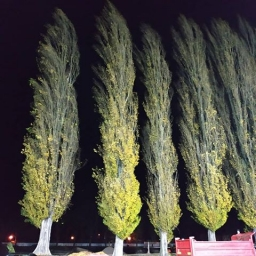Did you find an error or inaccuracy? Feel free to write us. Thank you!Tips to related online calculators
Need help to calculate sum, simplify or multiply fractions? Try our fraction calculator.
Do you have a linear equation or system of equations and looking for its solution? Or do you have a quadratic equation?
Do you want to convert length units?

## Related math problems and questions:

• Divisible by 5How many three-digit odd numbers divisible by 5, which are in place ten's number 3?
• From a 2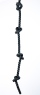From a rope that is 11 m long, two pieces of lengths 13/5 m and 33/10 m are cut off. What is the length of the remaining rope?
• PlayingHow long have we trained on the pitch when we know that the warm-up took 10 minutes, we trained passes for one-third of the time and we played football half the time?
• GardenThe garden around the new farm is divided as follows: 35% vegetable, 30% fruit orchards, 10% flowers and the remaining 120 m2 are lawns. What is the total area of the garden?
• Drawing from a hatWhen drawing numbers from a hat from 1 to 35, we select random given numbers. What is the probability that the drawn numbers will be divisible by 8 and 2?
• Lookout towerCalculate the height of a lookout tower forming a shadow of 36 m if at the same time a column 2.5 m high has a shadow of 1.5 m.
• Paper cuttingSusanna is cutting construction paper into rectangles for a project. She needs to cut one rectangle that is 10 inches × 14 1/2 inches. She needs to cut another rectangle that is 10 1/3 inches by 10 1/2 inches. How many total square inches of construction
• Iron pole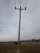The iron pole is in the ground 2/5 of its length, partly above the ground 1/3 is yellow, and the unpainted section is 6 m long. How long is the entire column?The nine-meter poplar casts a shadow 16.2 m long. How long does a shadow cast by Peter at the same time, if it is 1.4 m high?
• What is 9What is the value of x in the proportion 2 and one-fourth over x = 1 and one-half over 3 and three-fifths? 2 and two-fifths 5 and two-fifths 8 and 1 over 10 12 and 3 over 20
• Broken treeThe tree was 35 meters high. The tree broke at the height of 10 m above the ground. Top but does not fall off it refuted on the ground. How far from the base of the tree lay its peak?
• The swing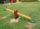To swing the two girls. Aneta weight 45 kg and Simon 35 kg weight. How far should sit Simon from the middle of swing so it is balanced, if we know that Aneta is sitting at distance 1,5m? How far are girls sitting apart?
• MilimetersThe pool is 6 meters long, 3 meters wide and the water in it is filled with water to a height 1.7 m. When John jumped into it and completely submerged, the level has risen by 5.4 mm. How much weight John when we know that one liter of the human body weigh
• Two rectangular boxesTwo rectangular boxes with dimensions of 5 cm, 8 cm, 10 cm, and 5 cm, 12 cm, 1 dm are to be replaced by a single cube box of the same cubic volume. Calculate its surface.
• Summerjob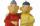Albert, Beny, Cyril and Dan worked on summerjob. From the total revenue Albert received 2/5, Beny 1/6 , 3/10 Cyril and Dan the rest which is 324 euros. How many euros earned every one of them?
• Swimming pool 5A rectangular inflatable swimming pool is 3 yards long, 2 5/8 yards wide, and  1 3/5 yard tall. What is the volume of the pool? Round to the nearest tenth.
• IS trapezoid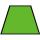Isosceles trapezoid arm measured 35 cm. Height is 30 cm and middle segment is 65 cm. Determine length of its bases.